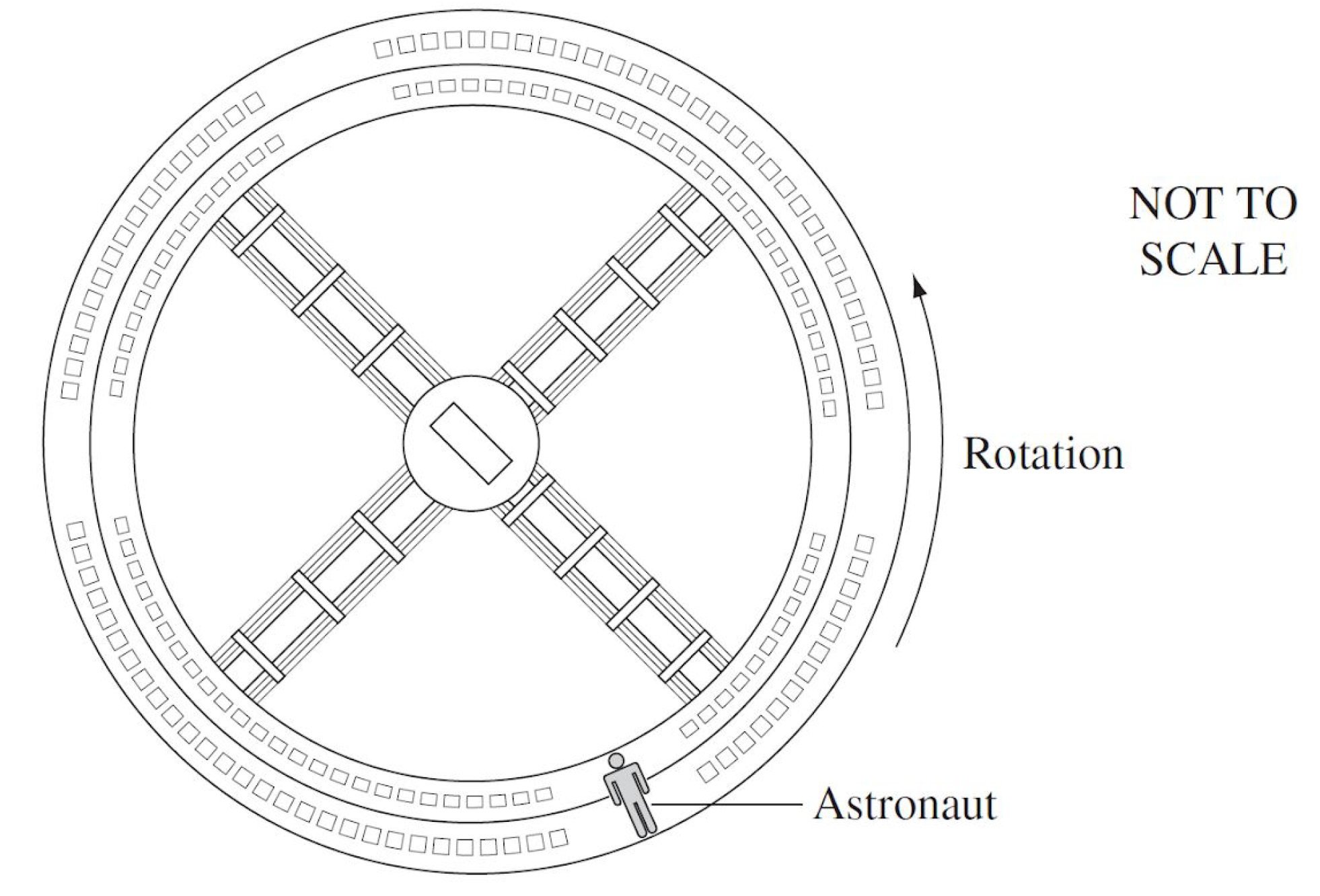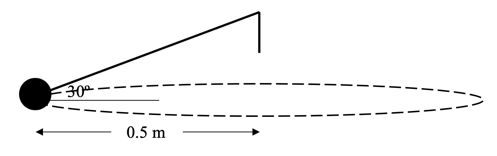# M5-S7: Quantitative Analysis of Circular Motion

• ### solve problems, model and make quantitative predictions about objects executing uniform circular motion in a variety of situations, using the following relationships:

- a_c=v^2/r
- v=(2pir)/T
- F_c=(mv^2)/r
- omega=(Deltatheta)/t

• Tangential velocity v is defined as the total circumference of circular path divided by the total time taken to complete one revolution T, which is also known as the period of circular motion.

v=(2pir)/T

• Angular velocity w is defined as the total angle of one revolution, 2p, divided by the period, T, of circular motion. Its SI unit is radians per second (rad s-1)
omega=(2pi)/T

v=omegar

Alternatively, angular velocity is also defined as the angle completed by an object in circular motion in time t, where t does not necessarily need to be the period of circular motion.

omega=(Deltatheta)/tFigure shows the direction of tangential and angular velocity.  vector points perpendicular to the v vector, can be determined by curling the right hand into a fist form with thumb pointing outwards. Curled fingers represent direction of rotation while thumb points in the direction of angular velocity.

Substituting  into centripetal force equation (F_c=(mv^2)/r) gives F_c=mromega^2 .

Derivation:

F_c=(m(omegar)^2)/r

F_c=(momega^2r^2)/r

F_c=mromega^2

## Practice Question 1 (NESA Sample Question)

A 15-gram metal ball bearing on a string is swung around a pole in a circle of radius 0.8 m. The plane of the circular path is horizontal. The angular velocity of the motion is 4pi   rad s^-1.What is the magnitude of the centripetal force required to maintain the motion of the ball?

## Practice Question 2

A particle is moving around in a circle of radius 1.5 m with a constant speed of 2 ms-1. Calculate its

(a) centripetal acceleration
(b) angular velocity

## Practice Question 3 (HSC 2013)

The diagram shows a futuristic space station designed to simulate gravity in a weightless environment.If the space station has a diameter of 550 m, calculate the rotational speed needed to simulate 1g of gravitational acceleration.

## Practice Question 4

A 15 kg weight is attached to a rope and spun in uniform circular motion with a 0.5 m radius as shown.If the rope makes an angle of 30º with the horizontal during circular motion, calculate the angular velocity of the weight.

## Solutions

Practice Question 1

F_c=mromega^2

Convert grams into SI unit (kg):

F_c=(15/1000)(0.8)(4pi)^2

F_c=1.9 N

Practice Question 2

(a) We will use a_c=v^2/r to find centripetal acceleration:

a_c=(2)^2/1.5

a_c=2.7  ms^-2 towards the centre of the circle

(b) We will use omega=v/r to find angular velocity:

omega=2/1.5

omega=1.3   rad s^-1

Practice Question 3
It is important to first recognise the source of centripetal force in this scenario. You can read more about it in Introduction to Circular Motion.
We will use a_c=v^2/r to calculate the rotation speed (tangential). Since diameter equals 550 m, radius is 550/2 = 275.

9.8 = v^2/275

v^2=275xx9.8

v=sqrt(275xx9.8)

v=51.9   ms^-1
Practice Question 4
This question is not as intuitive as the others. To calculate the angular velocity and tension force, we need to analyse the forces in vertical and horizontal plane.Since there is no motion in the vertical plane, the vertical component of tension force (T_y) equals to the weight's weight force (mg) in magnitude.

Tsin30º=mg

Tsin30º=(2.00)(9.8)

T=(2.00xx9.8)/(sin30º)

T=39.2 N towards the centre of circular motion

Now, the horizontal component of tension force provides the centripetal force for circular motion. So:

F_c=Tcos30º

(mv^2)/r=Tcos30º

((2.00)v^2)/0.5=(39.2)cos30º

v^2=(0.5xx39.2xxcos30º)/2.00

v=sqrt((0.5xx39.2xxcos30º)/2.00)

v=2.91   ms^-1

Using tangential velocity v, we can now find the angular velocity:

omega=v/r

omega=2.91/0.5

omega=5.83   rads^-1

Next section: Torque